# numpy 学习 第2篇：ndarray 基础操作

numpy模块内置的函数能够对数组进行复杂而高效的操作，这些函数中都有一个参数axis（轴）。在数组中，轴表示维度，对于二维数组，axis参数的取值通常有：

• 当axis为None，表示把数组展开为一维数组；
• 当axis为0时，表示按照列（第一维）进行计算；
• 当axis=1时，表示按照行（第二维）进行计算。

## 一，排序sort

sort(axis,kind)函数用于对数组进行排序，可以使用类方法numpy.sort()，返回的是数组的已排序的副本，而原始数组并没有改变；也可以使用对象方法obj.sort()，原始数组排序。

numpy.sort(a, axis=1, kind='quicksort')

• a：所需排序的数组
• axis：数组排序时的轴，axis=0排序；axis=1按行排序，默认值是1
• kind：数组排序时使用的方法，其中：kind= ′quicksort ′为快排；kind=′mergesort′ 为归并排序；kind=′heapsort′为堆排
• axis指定排序的轴；kind指定排序算法，默认的排序算法是快速排序，np.sort()返回的是数组的已排序的副本，而就是排序则会修改数组本身。

import numpy as np

a = np.array([[1, 2, 1]
,[1,1,0]])

r1=np.sort(a)
r2=np.sort(a,axis=0)
r3=np.sort(a,axis=1)

print('a.sort() = {0}\na.sort(axis=0) = {1}\na.sort(axis=1) ={2}'.format(r1,r2,r3))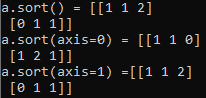## 二，argsort()函数

argsort()函数返回的是按照数组值从小到大的索引值，即返回的是索引值，索引值是按照元素值从小到大得到的。

x=np.array([1,4,3,-1,6,9])
y=np.argsort(x)
#output
array([3, 0, 2, 1, 4, 5], dtype=int64)

argsort()函数是将x中的元素从小到大排列提取其对应的index(索引)，然后输出到y，按照降序排序：

y=np.argsort(-x)

y=np.argsort(x)

## 三，vectorize 向量化

numpy.vectorize()函数定义一个向量化函数，它以序列或numpy数组作为输入，对numpy数组做向量化操作。函数输出的数据类型是由otypes参数确定的，如果otypes=None，那么输出的数据类型是通过使用输入的第一个元素调用函数来确定的，该函数返回一个函数对象。

numpy.vectorize(pyfunc, otypes=None, doc=None, excluded=None, cache=False, signature=None)

• pyfunc：函数对象
• otype：输出数据的类型（putput data type）

def myfunc(a, b):
return a-b if a>b else a+b

vfunc = np.vectorize(myfunc)
vfunc([1, 2, 3, 4], 2)
#output array([3, 4, 1, 2])

## 四，reshap

reshap(array,newshape) ：返回一个给定shape的数组的副本，例如，下面的代码把一个一维数组转换为4行2列的二位数组：

a=np.arange(8)
np.reshape(a,(4,2))

## 五，flatten

a=np.arange(8)
b=np.reshape(a,(4,2))
b.flatten()

## 六，计算元素的和/积

numpy计算元素的和/积，在语法上相似。

1，求和

numpy对象的函数：sum(axis)

• 当参数为None时，把二维数组中的所有元素相加；
• 当参数为0时，把二维数组中，按照列对元素相加；
• 当参数为1时，把二维数组中，按照行对元素相加；

import numpy as np

a = np.array([[0, 2, 1]
,[0,1,0]])

r1=a.sum()
r2=a.sum(axis=0)
r3=a.sum(axis=1)

print('a.sum() = {0}\na.sum(axis=0) = {1}\na.sum(axis=1) ={2}'.format(r1,r2,r3))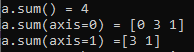2，求积

• 当参数为None时，把二维数组中的所有元素相乘；
• 当参数为0时，把二维数组中，按照列对元素相乘；
• 当参数为1时，把二维数组中，按照行对元素相乘；

import numpy as np

a = np.array([[1, 2, 1]
,[1,1,0]])

r1=a.prod()
r2=a.prod(axis=0)
r3=a.prod(axis=1)

print('a.prod() = {0}\na.prod(axis=0) = {1}\na.prod(axis=1) ={2}'.format(r1,r2,r3))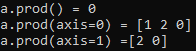## 七，计算统计量

• mean(axis)：计算元素的均值
• var(axis)：计算元素的方差
• std(axis) ：计算元素标准差
• max(axis)：计算元素的最大值
• min(axis)：计算元素的最小值
• ptp(axis)：计算元素的取值范围，即最大值和最小值的差值
• median(axis)：计算元素的中位数

import numpy as np

a = np.array([[1, 2, 1]
,[1,1,0]])

r1=a.mean()
r2=a.mean(axis=0)
r3=a.mean(axis=1)

print('a.mean() = {0}\na.mean(axis=0) = {1}\na.mean(axis=1) ={2}'.format(r1,r2,r3))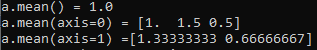## 八，最大值或最小值的索引

• argmax(axis)：返回最大值的索引
• argmin(axis)：返回最小值的索引

import numpy as np

a = np.array([[1, 2, 1]
,[1,1,0]])

r1=a.argmin()
r2=a.argmin(axis=0)
r3=a.argmin(axis=1)

print('a.argmin() = {0}\na.argmin(axis=0) = {1}\na.argmin(axis=1) ={2}'.format(r1,r2,r3))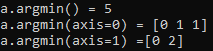## 九，等差数列

numpy.linspace(start, stop[, num=50[, endpoint=True[, retstep=False[, dtype=None]]]]])

• start - 起始点，
• stop - 结束点
• num - 元素个数，默认为50，
• endpoint - 是否包含stop数值，默认为True，包含stop值；若为False，则不包含stop值
• retstep - 返回值形式，默认为False，返回等差数列组，若为True，则返回结果(array([samples, step])),
• dtype - 返回结果的数据类型，默认无，若无，则参考输入数据类型。
>>> import numpy as np
>>> np.linspace(-3,3,11)
array([-3. , -2.4, -1.8, -1.2, -0.6,  0. ,  0.6,  1.2,  1.8,  2.4,  3. ])

## 十，分箱

numpy.digitize(data,bins)

## 十一，随机数生成

numpy.random模块是对Python内置的random的升级，增加了一些用于搞笑生成多种概率分布的样本值的函数。

• seed：确定随机数生成器的种子
• permutation：返回一个序列的随机排列
• shuffle：对一个序列就地进行随机排列
• rand：产生均匀分布的样本值
• randint：从给定的上下限范围内随机选取正数
• randn：产生标准正态分布
• binomial：产生二项分布的样本值
• normal：产生高斯正态分布的样本值
• beta：产生Beta分布的样本值
• chisquare：产生卡方分布的样本值
• gamma：产生伽马分布的样本值
• uniform：产生在[0,1]范围中均匀分布的样本值

samples=np.random.normal(size=(4,4))

numpy中sum（axis=0）和axis=1的计算原理

posted @ 2019-10-10 10:35 悦光阴 阅读(...) 评论(...) 编辑 收藏